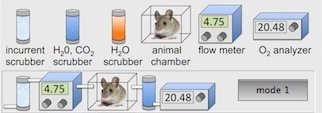Gas exchange:   VO2 calculation example File Edit Respirometry Analyze View Script Window Special

This example shows how to derive the equation for converting flow rate and the animal-induced change in oxygen concentration into the rate of oxygen consumption (VO2, ml/min).   Please assume that the V symbols represent rates (and therefore should have a dot over the V, if I knew how to do that in html).
The arrangement of the respirometry system is the LabAnalyst 'Mode 1' configuration, with the flowmeter upstream from the animal chamber and both CO2 and water vapor absorbed before O2 concentration is measured:Obviously VO2 is simply the difference between the amount of O2 entering the metabolism chamber and the amount of O2 leaving the chamber (all in ml/min):
(1)          VO2   =  O2in - O2out

More specifically:

O2in   =  FLOWin * FiO2       and      O2out   =  FLOWout * FeO2

Where FLOWin is the incurrent air flow into the chamber (ml/min), FiO2 is the concentration of O2 in incurrent air (usually .2095), FLOWout   =  the excurrent air flow out of the chamber (ml/min), and FeO2 is the O2 concentration in excurrent air.

In the Mode 1 arrangement, FLOWin is measured, but FLOWout is not.   But we can calculate FLOWout as:

FLOWout   =  FLOWin + VCO2 + VH2O - VO2

Where VCO2 is CO2 production by the animal and VH2O is water loss from the animal (both in ml/min).  Since the Mode 1 arrangement uses scrubbers to remove both water and CO2 from excurrent air:

FLOWout   =  FLOWin - VO2

Accordingly, we can re-write equation (1) as:

(2)         VO2   =  FLOWin * FiO2   -   (FLOWin - VO2) * FeO2

rearranging:

(3)         VO2   =  FLOWin * FiO2  -   FLOWin * FeO2   +   VO2 * FeO2

and:

(4)         VO2   =  FLOWin * (FiO2 - FeO2)   +   VO2 * FeO2

rearranging again:

(5)         VO2   -   (VO2 * FeO2)   =  FLOWin * (FiO2 - FeO2)

(6)         VO2 * (1 - FeO2)   =  FLOWin * (FiO2 - FeO2)

and finally:

(7)         VO2   =  FLOWin * (FiO2 - FeO2) / (1 - FeO2)
Reminder:   all of the V symbols should have a dot over the letter V, indicating a rate instead of a volume.